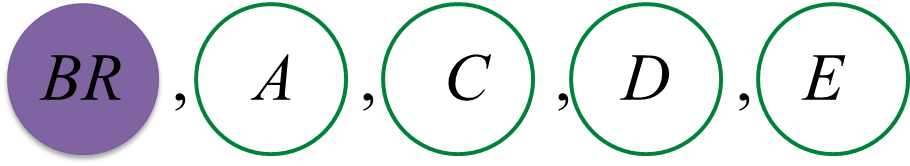\

# SPM Additional Mathematics 2017, Paper 1 (Question 20 – 22)

Question 20 (4 marks):
Table 1 shows the distribution of marks for 40 students in an Additional Mathematics test. The number of students for the class interval 40 – 59 is not stated.Table 1

(a) State the modal class.
(b) Puan Zainon, the subject teacher, intends to give a reward to the top ten students. Those students who achieve the minimum mark in the top ten placing will be considered to receive the reward. Elina obtains 74 marks.
Does Elina qualify to be considered to receive the reward? Give your reason.

Solution:
(a)
4 + 10 + x + 8 + 7 = 40
x + 29 = 40
x = 11

Modal class = 40 – 59

(b)

Question 21 (3 marks):
A biased cube dice is thrown. The probability of getting the number ‘4’ is $\frac{1}{16}$  and the probabilities of getting other than ‘4’ are equal to each other.
If the dice is thrown twice, find the probability of getting two different numbers.

Solution:

Question 22 (4 marks):
Danya has a home decorations shop. One day, Danya received 14 sets of cups from a supplier. Each set contained 6 pieces of cups of different colours.

(a)
Danya chooses 3 sets of cups at random to be checked.
Find the number of different ways that Danya uses to choose those sets of cups.

(b)
Danya takes a set of cups to display by arranging it in a row.
Find the number of different ways the cups can be arranged such that the blue cup is not displaced next to the red cup.

Solution:
(a)
Number of different ways 3 sets of cups at random to be checked
= 14C3
=364

(b)Number of ways (Blue cup and red cup are next to each other)
= 5! × 2!
= 240

Number of different ways the cups can be arranged such that the blue cup is not displaced next to the red cup
= 6! – 240
= 720 – 240
= 480